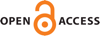Download this articleFor screen For printingRecent IssuesThe Journal About the Journal Editorial Board Editors’ Interests Subscriptions Submission Guidelines Submission Form Policies for Authors Ethics Statement ISSN: 1948-206X (e-only) ISSN: 2157-5045 (print) Author Index To Appear Other MSP JournalsSubelliptic wave equations are never observable

### Cyril Letrouit

Vol. 16 (2023), No. 3, 643–678##### Abstract

It is well known that observability (and, by duality, controllability) of the elliptic wave equation, i.e., with a Riemannian Laplacian, in time ${T}_{0}$ is almost equivalent to the geometric control condition (GCC), which stipulates that any geodesic ray meets the control set within time ${T}_{0}$. We show that in the subelliptic setting, the GCC is never satisfied, and that subelliptic wave equations are never observable in finite time. More precisely, given any subelliptic Laplacian $\mathrm{\Delta }=-{\sum }_{i=1}^{m}{X}_{i}^{\ast }{X}_{i}$ on a manifold $M$, and any measurable subset $\omega \subset M$ such that $M\setminus \omega$ contains in its interior a point $q$ with $\left[{X}_{i},{X}_{j}\right]\left(q\right)\notin \mathrm{Span}\left({X}_{1},\dots ,{X}_{m}\right)$ for some $1\le i,j\le m$, we show that, for any ${T}_{0}>0$, the wave equation with subelliptic Laplacian $\mathrm{\Delta }$ is not observable on $\omega$ in time ${T}_{0}$.

The proof is based on the construction of sequences of solutions of the wave equation concentrating on geodesics (for the associated sub-Riemannian distance) spending a long time in $M\setminus \omega$. As a counterpart, we prove a positive result of observability for the wave equation in the Heisenberg group, where the observation set is a well-chosen part of the phase space.

##### Keywords
subelliptic, wave equation, observability, sub-Riemannian
##### Mathematical Subject Classification 2010
Primary: 22E25, 35H20, 35L05, 93B05, 93B07
Secondary: 35H10, 35S05, 78A05
##### Milestones